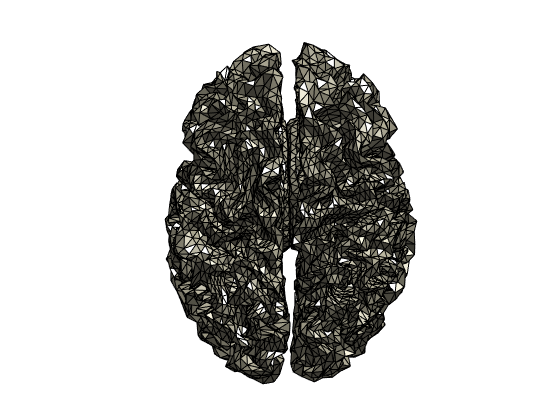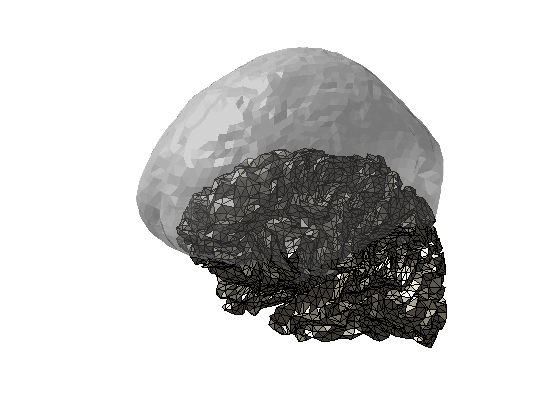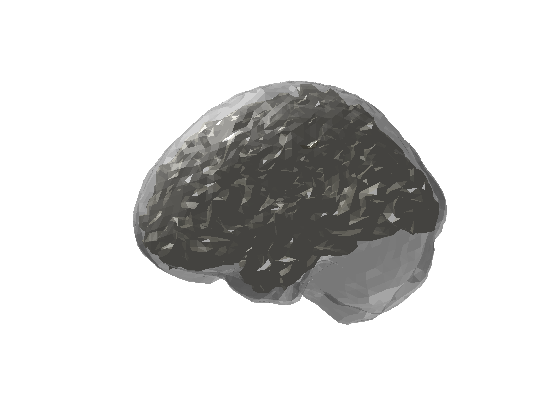### Set up general paths

Change these to appropriate paths for your operating system and setup

``````%% paths

ft_defaults

raw_meg_path = '/archive/20067_workshop_source_reconstruction/MEG/';
meg_path = '/home/share/workshop_source_reconstruction/data/MEG/';
mri_path = '/home/share/workshop_source_reconstruction/data/MRI/';``````

### Set up subject specific paths

Make subject and recording specific paths (the cell array “subjects_and_dates” can be expanded)

``````%% subjects and dates

subjects_and_dates = ...
{
'NatMEG_0177/170424/'
};

output_path = fullfile(meg_path, subjects_and_dates{1});
cd(output_path)

events = [1 2 4 8 16]; %% right little, right ring, right middle, right index, right thum
``````

## Import source space

Minimum-norm estimate (MNE) use a cortical surface as source model. In the tutorial documentation “Prepare source space for MNE” (http://natmeg.se/onewebmedia/prepare_MNE_sourcespace.html) you can read how to export MRI to Freesurfer to extract the cortical surface and setup a source model with MNE-C. This creates a set of point equally sampled across the cortical surface that is used for source modeling.

You will find the file “Sub02-oct-6-src.fif” in the tutorial datafiles.

``sourcemodel = ft_read_headshape('Sub02-oct-6-src.fif', 'format', 'mne_source'); ``
• What does the “source model” structure contain?

Note that the units are in meters. You can use ft_convert_units to make the units milimeters instead:

``sourcemodel = ft_convert_units(sourcemodel, 'mm');``

You can plot the source model with the function ft_plot_mesh:

``figure; ft_plot_mesh(sourcemodel, 'edgecolor', 'k'); camlight ``Looks nice!

Each point on the grid will represent a dipole in the MNE source reconstruction.

• How many dipoles will our MNE source reconstruction contain?

``````load headmodel_mne.mat
``````

Now you should have the three main ingredients for doing MNE source reconstruction with MEG:

1. Processed evoked MEG data.
2. A volume model of the brain.
3. A source space model of the cortical surface.

Take a look at the headmodel and sourcemodel toghether:

``````figure; hold on
ft_plot_mesh(sourcemodel, 'edgecolor', 'k'); camlight
``````• What is going on?

### Aligning source space and volume model

We have aligned the MRI that we used to create the volume model to the Neuromag coordinate system used by the MEG scanner. However, in order to create the cortical surface in Freesurfer, we had to convert the MRI to the spm coordinate system and exported it to Freesurfer (see http://natmeg.se/onewebmedia/prepare_MNE_sourcespace.html). When we imported the source space back into MATLAB, it kept this coordinate system. Our head model and source space are now in two different coordinate systems.

To get the cortical surface back into the head, you need to transform the points in source space to the coordinate system of the head model. Load the transformation matrix of the resliced MRI in the spm coordinate system. We will use this to create a transformation from the spm coordinate system to the neuromag coordinate system. If you have run the prepare MNE source space tutorial, you must read the transformation files you created yourself.

``````% from voxel to spm-coordsys.

% from voxel to neuromag-coordsys.
transform_vox2neuromag = mri_segmented_mne.transform;

% Get transformation matrix from spm to neuromag
T = transform_vox2neuromag/transform_vox2spm_rs;``````

Now transform the source space using the transformation matrix T. Note, that if you run the following line several times, it will apply the transformation each time, transforming from the current position to a new position, which will be wrong. If in doubt, save the source model before proceeding.

``sourcemodel = ft_transform_geometry(T, sourcemodel);``

Take a look at the headmodel and sourcespace again:

``````figure; hold on
ft_plot_mesh(sourcemodel, 'edgecolor', 'k'); camlight
``````Remember to save the source model.

``````save('sourcemodel.mat','sourcemodel')
disp('Done');``````

## MNE source reconstruction on MEG data

Now we can create the leadfield and do the source reconstruction. Load the relevant files (if you do not already have then loaded):

``````load timelockeds.mat

For this tutorial we will use the stimulation of the indexfinger – corresponding the the 4th condition:

``data_meg = timelockeds{4};``

``````
cfg = [];
cfg.channel             = 'meggrad';                  % the used channels
cfg.senstype            = 'meg';
cfg.grid.pos            = sourcemodel.pos;            % source points
cfg.grid.inside         = 1:size(sourcemodel.pos,1);  % all source points are inside of the brain

• Take a look at the “leadfield_mne” structure. How does it compare to the leadfield stucturs you created for the dipole tutorial? Hint: look at the size of the “pos”" matrix or try to plot the points:
``````plot3(leadfield_mne.pos(:,1),leadfield_mne.pos(:,2),leadfield_mne.pos(:,3),'0')

Finally, we do the source reconstruction using ft_sourceanalysis. Specify cfg.method = ‘mne’ to use MNE. MNE also require additional parameters indicating how to scale the noise covariance. Note that the noise covariance matrix already is in the “data” structure. This was calculated already in the preprocessing steps when the evoked fields were calculated.

``````cfg                     = [];
cfg.method              = 'mne';
cfg.senstype            = 'meg';
cfg.mne.prewhiten       = 'yes';
cfg.mne.lambda          = 3;
cfg.mne.scalesourcecov  = 'yes';
source_mne  = ft_sourceanalysis(cfg,data_meg);

save source_mne.mat source_mne;
disp('Done')``````
• Look in the source_mne structure – especially the source_mne.avg structure. What do we get?

## Visualize

Plot the MNE source reconstruction on the grid. The source_mne structure contains values for all grid points and all timepoints. To visualize the source reconstruction on the grid, we need to decide what time points to plot.

Go back to the evoked data to find some interesting times to plot using ft_multiplotER. Since we used the gradiometers, we will start by plotting the fields from the combined gradiometers. For example, let us compare the first peak around 50 ms and the larger peak from 140-150 ms. Use the drag and click tool on the multiplot to plot topographical plots.

``````data_cmb = ft_combineplanar([],data_meg); %Combine gradiometers

cfg = [];
cfg.layout = 'neuromag306cmb.lay';
figure; ft_multiplotER(cfg, data_cmb);``````
• Find peaks in sensor space, look at the topographies and try to guess what the sources might look like?